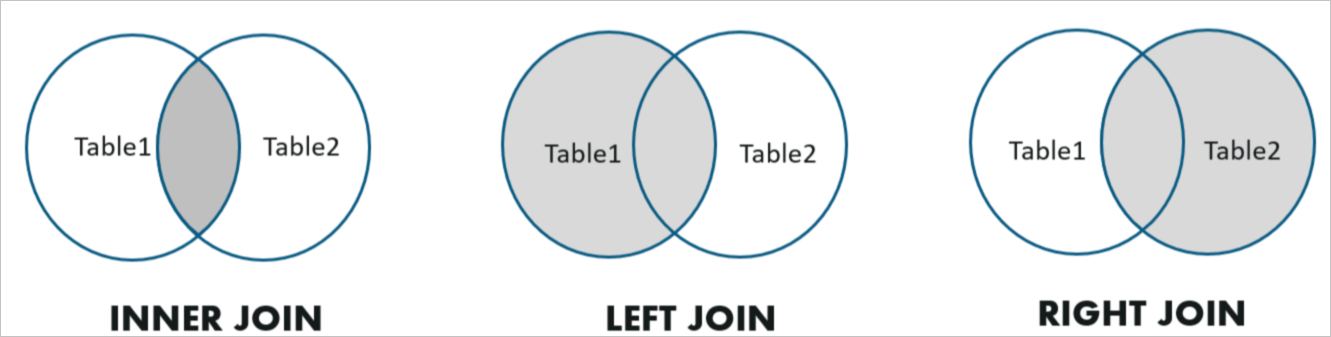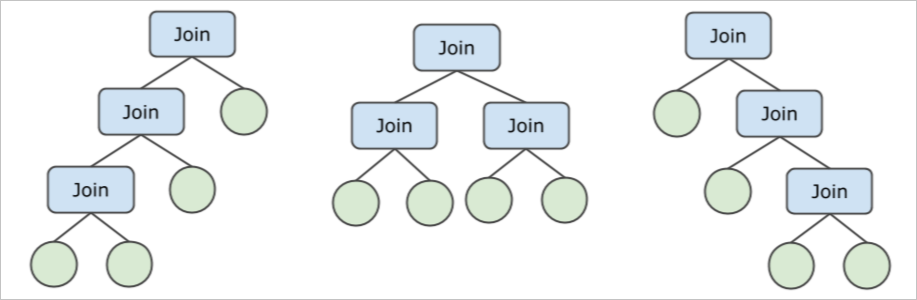This topic describes how the optimizer and executor of PolarDB-X process JOIN operations. A JOIN operation is an operation that combines rows from multiple tables based on one or more common columns among these tables.

## What is a JOIN operation?

JOIN operations are commonly used in SQL queries. The semantics of JOIN is equivalent to calculating the Cartesian product of two tables and then retaining the data that meets the filter conditions. In most cases, equi joins are used. An equi join is used to combine two tables based on the values of a specified column.

A subquery is a query nested inside another SQL query. The result of a subquery is passed to the outer query and then used to process the outer query. Subqueries can be used in different components of an SQL statement. For example, a subquery can be used as the output data in a SELECT clause, an input view in a FROM clause, or a filter condition in a WHERE clause.

The JOIN operations described in this topic cannot be pushed down. MySQL at the storage layer determines how to execute the JOIN operations that can be pushed down to LogicalView.

## Types of JOIN operations

PolarDB-X supports the following common JOIN operation types: inner joins, left outer joins, and right outer joins.The following examples show different types of JOIN operations:
``````/* Inner Join */
SELECT * FROM A, B WHERE A.key = B.key;
/* Left Outer Join */
SELECT * FROM A LEFT JOIN B ON A.key = B.key;
/* Right Outer Join */
SELECT * FROM A RIGHT OUTER JOIN B ON A.key = B.key;``````
PolarDB-X also supports semi joins and anti joins. Semi joins and anti joins cannot be written in SQL. They are implemented by using subqueries nested inside an EXISTS or IN condition. The following code blocks provide examples of semi joins and anti joins:
``````/* Semi Join - 1 */
SELECT * FROM Emp WHERE Emp.DeptName IN (
SELECT DeptName FROM Dept
)
/* Semi Join - 2 */
SELECT * FROM Emp WHERE EXISTS (
SELECT * FROM Dept WHERE Emp.DeptName = Dept.DeptName
)
/* Anti Join - 1 */
SELECT * FROM Emp WHERE Emp.DeptName NOT IN (
SELECT DeptName FROM Dept
)
/* Anti Join - 2 */
SELECT * FROM Emp WHERE NOT EXISTS (
SELECT * FROM Dept WHERE Emp.DeptName = Dept.DeptName
)``````

## Join algorithms

PolarDB-X supports multiple join algorithms, such as nested loops join, hash join, sort-merge join, and lookup join. Lookup join is also known as BKA join.

Nested-Loop Join (NLJoin)

Nested loops joins are commonly used for non-equi joins. A nested loops join works in the following way:
1. Selects all rows from the inner table and caches the rows in memory. The inner table refers to the right table that contains less data in most cases.
2. Scans the entire outer table. For each row in the outer table:
• Each row is mapped to a row of the inner table cached in memory.
• Creates a result set, checks whether the result set meets the join condition, and returns the result set if the condition is met.
The following code block provides an example of a nested loops join:
``````> EXPLAIN SELECT * FROM partsupp, supplier WHERE ps_suppkey < s_suppkey;

NlJoin(condition="ps_suppkey < s_suppkey", type="inner")
Gather(concurrent=true)
LogicalView(tables="partsupp_[0-7]", shardCount=8, sql="SELECT * FROM `partsupp` AS `partsupp`")
Gather(concurrent=true)
LogicalView(tables="supplier_[0-7]", shardCount=8, sql="SELECT * FROM `supplier` AS `supplier`")``````
In most cases, nested loops join is the least efficient join algorithm. Nested loops joins can be used only in the following scenarios: The join is a non-equi join as shown in the preceding example or the inner table contains a small amount of data. You can use the following hint to force PolarDB-X to use nested loops joins and specify the join order:
``/*+TDDL:NL_JOIN(outer_table, inner_table)*/ SELECT ...``
You can also use the result of joining multiple tables as the inner_table or outer_table, as shown in the following example:
``/*+TDDL:NL_JOIN((outer_table_a, outer_table_b), (inner_table_c, inner_table_d))*/ SELECT ...``

Hash Join

Hash join is one of the most commonly used join algorithms for equi joins. A hash join works in the following way:
• Selects all rows from the inner table and writes rows into a hash table stored in memory. The inner table refers to the right table that contains less data in most cases.
• Scans the entire outer table. For each row in the outer table:
• Scans the hash table against the join key in the equality condition and selects 0 to N rows that have the same join key.
• Creates a result set, checks whether the result set meets the join condition, and returns the result set if the condition is met.
The following code block provides an example of hash joins:
``````> EXPLAIN SELECT * FROM partsupp, supplier WHERE ps_suppkey = s_suppkey;

HashJoin(condition="ps_suppkey = s_suppkey", type="inner")
Gather(concurrent=true)
LogicalView(tables="partsupp_[0-7]", shardCount=8, sql="SELECT * FROM `partsupp` AS `partsupp`")
Gather(concurrent=true)
LogicalView(tables="supplier_[0-7]", shardCount=8, sql="SELECT * FROM `supplier` AS `supplier`")``````

Hash joins are commonly used in complex queries that join large amounts of data but cannot be optimized by index lookup. In this case, hash join is the optimal algorithm. In the preceding example, the system must perform full table scans on the partsupp and supplier tables. This involves large amounts of data. Therefore, hash joins are suitable for this scenario. The inner table of a hash join is used to create a hash table stored in memory. Ensure that the inner table contains less data than the outer table. In most cases, the optimizer can automatically choose the optimal join order.

You can also use the following hint to force PolarDB-X to use hash joins and specify the join order:
``/*+TDDL:HASH_JOIN(table_outer, table_inner)*/ SELECT ...``

Lookup Join (BKAJoin)

Lookup join is another commonly used join algorithm for equi joins and is commonly used in scenarios where a small amount of data is involved. A lookup join works in the following way:
1. Scans the entire outer table. The outer table refers to the left table that contains less data in most cases. For each batch (for example, every 1,000 rows) from the outer table:
2. Creates an IN (....) condition that uses the join key of the rows in the batch, and then adds the condition to the inner table query.
3. Executes the inner table query to select the rows that meet the join condition.
4. Maps each row in the outer table to a row in the inner table based on a hash table, merges the rows from the inner and outer tables, and then returns the result.
The following code block provides an example of lookup joins (BKA joins):
``````> EXPLAIN SELECT * FROM partsupp, supplier WHERE ps_suppkey = s_suppkey AND ps_partkey = 123;

BKAJoin(condition="ps_suppkey = s_suppkey", type="inner")
LogicalView(tables="partsupp_3", sql="SELECT * FROM `partsupp` AS `partsupp` WHERE (`ps_partkey` = ?)")
Gather(concurrent=true)
LogicalView(tables="supplier_[0-7]", shardCount=8, sql="SELECT * FROM `supplier` AS `supplier` WHERE (`s_suppkey` IN ('?'))")``````

Lookup joins are suitable if the outer table contains a small amount of data. In the preceding example, only a few rows are selected from the left table partsupp due to the filter condition ps_partkey = 123. In addition, the s_suppkey IN ( ... ) condition matches the primary key index of the right table. This reduces the cost of the lookup join.

You can use the following hint to force PolarDB-X to use lookup joins and specify the join order:
``/*+TDDL:BKA_JOIN(table_outer, table_inner)*/ SELECT ...``
Note The inner table of a lookup join must be a single table instead of the result of joining multiple tables.

Sort-Merge Join

Sort-merge join is another join algorithm for equi joins. A sort-merge join is reliant on the orders of the input rows in the left and right tables. The input rows must be sorted based on the join key. A sort-merge join works in the following way:
1. Uses MergeSort or MemSort to sort the input rows.
2. Compares the input rows in the left and right tables by using the following methods:
• Matches the current right row against the next left row If the join key of the current left row is smaller than that of the current right row.
• Matches the current left row against the next right row If the join key of the current right row is smaller than that of the current left row.
• Merges the left and right rows if the two rows have the same join key and the join condition is met, and then returns the result.
The following code block provides an example of lookup joins (BKA joins):
``````> EXPLAIN SELECT * FROM partsupp, supplier WHERE ps_suppkey = s_suppkey ORDER BY s_suppkey;

SortMergeJoin(condition="ps_suppkey = s_suppkey", type="inner")
MergeSort(sort="ps_suppkey ASC")
LogicalView(tables="QIMU_0000_GROUP,QIMU_0001_GROUP.partsupp_[0-7]", shardCount=8, sql="SELECT * FROM `partsupp` AS `partsupp` ORDER BY `ps_suppkey`")
MergeSort(sort="s_suppkey ASC")
LogicalView(tables="QIMU_0000_GROUP,QIMU_0001_GROUP.supplier_[0-7]", shardCount=8, sql="SELECT * FROM `supplier` AS `supplier` ORDER BY `s_suppkey`")``````

Pay attention to the MergeSort operator in the preceding execution plan and the ORDER BY operator that is pushed down. These operators are used to ensure that the input rows in the left and right tables of a sort-merge join are sorted based on the join key s_suppkey (ps_suppkey).

Sort-merge join is not the optimal join algorithm because it must sort input rows first. However, the user may want to sort the query result based on the join key, as shown in the preceding example. In this case, sort-merge join is the optimal choice.

You can use the following hint to force PolarDB-X to use sort-merge joins:
``/*+TDDL:SORT_MERGE_JOIN(table_a, table_b)*/ SELECT ...``

## Join order

In scenarios where multiple tables are joined, the optimizer must decide the order in which the tables are joined. This is because the join order affects the size of the intermediate result set and the cost of the execution plan.

For example, a JOIN operation is performed on four tables and the JOIN operation is not pushed down. In this case, the following join trees are applicable. In addition, the four tables can be sorted in up to 24 ways. As a result, up to 72 different join orders are available.PolarDB-X uses an adaptive strategy to create the optimal execution plan for a given JOIN operation that is performed on N tables.
• If the JOIN operation is not pushed down and the value of N is small, the bushy tree is used to create the optimal execution plan.
• If the JOIN operation is not pushed down and the value of N is large, the zig-zag or left-deep tree is used to create the optimal execution plan. This reduces the number of times of numerations and cost.

PolarDB-X uses a cost-based optimizer to select the join order that incurs the lowest cost. For more information, see Query optimizers.

In addition, different join algorithms have different preferences for the left and right tables. For example, the right table of a hash join is the inner table and is used to create a hash table. Therefore, specify the table that contains less data as the right table. The cost-based optimizer also has similar preferences.

PolarDB-X supports various join algorithms. The optimizer selects a proper join algorithm based on the collected statistics. The following table describes the scenarios where the join algorithms are applicable.
Join algorithm Scenario
NLJoin Non-equi joins.
HashJoin Hash join is used for most equi joins unless the data is severely skewed.
BKAJoin The outer table contains a small amount of data. The inner table contains a large amount of data.
Sort-Merge-Join The data is severely skewed or the data is already sorted.